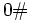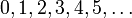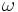# Difference between revisions of "Primorial"

## Definition

Letbe a natural number. Theprimorial, sometimes denoted, is defined as the product of the firstprime numbers.

The primorialis defined to be.

An alternate definition of primorial, called here the primorial of the second kind, is defined as the product of all the primes less than or equal to a given number. Note that the logarithm of the primorial of the second kind is the first Chebyshev function.

## Occurrence

### Initial values

The values of primorials atare given in the list: 1, 2, 6, 30, 210, 2310 View list on OEIS

## Special properties

• The primorialis the smallest natural numberwith, whereis the prime divisor count function.
• Every primorial is a minimum-so-far for the ratio of the Euler phi-function and the identity function.